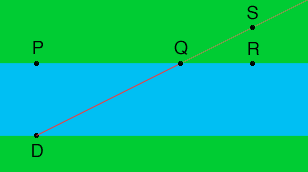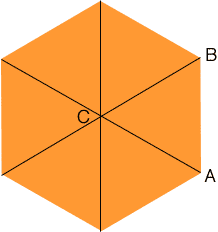Quandaries and Queries Hi My name is Sara I am a substitute teacher for all grade levels. I had a couple of math problems to do with the 10th grade math class. I hope that you can help. #1 A boat is traveling in a straight line across a river from point D, directly opposite town P, to a point Q, two thirds of the distance from town P to town R. Towns P and R are towns on the same side of the lake and are 18 miles apart. Line DQ passes through town S, which is 8 miles north of town R. Points P, Q, and R are collinear. What is the length of QS in Miles? How many miles does the boat travel across the river? #2 What is the area of a regular hexagon if the perimeter is 24cm? Each side is 4cm right? Hi Sara, Below is my diagram for the first problem.The distance from P to R is 18 miles and Q is two thirds the way from P to R, so Q is 12 miles from P and hence the distance from Q to R is 6 miles. The distance from R to S is 8 miles and hence SQR is a right triangle with sides of length 8 miles and 8 miles. What is the length of the hypotenuse? The key to the second problem is also to draw a diagram.I joined the pairs of opposite vertices of the hexagon to form six, congruent triangles which share a common vertex at C. Since the sum of the six angles at C is 360o, each of the angles at C is measures 60o. Consider one of these triangles ABC. It is an isosceles triangle, its base is 4 cm in length, and the angle at its apex measures 60o. Can you find its area? Penny Go to Math Central